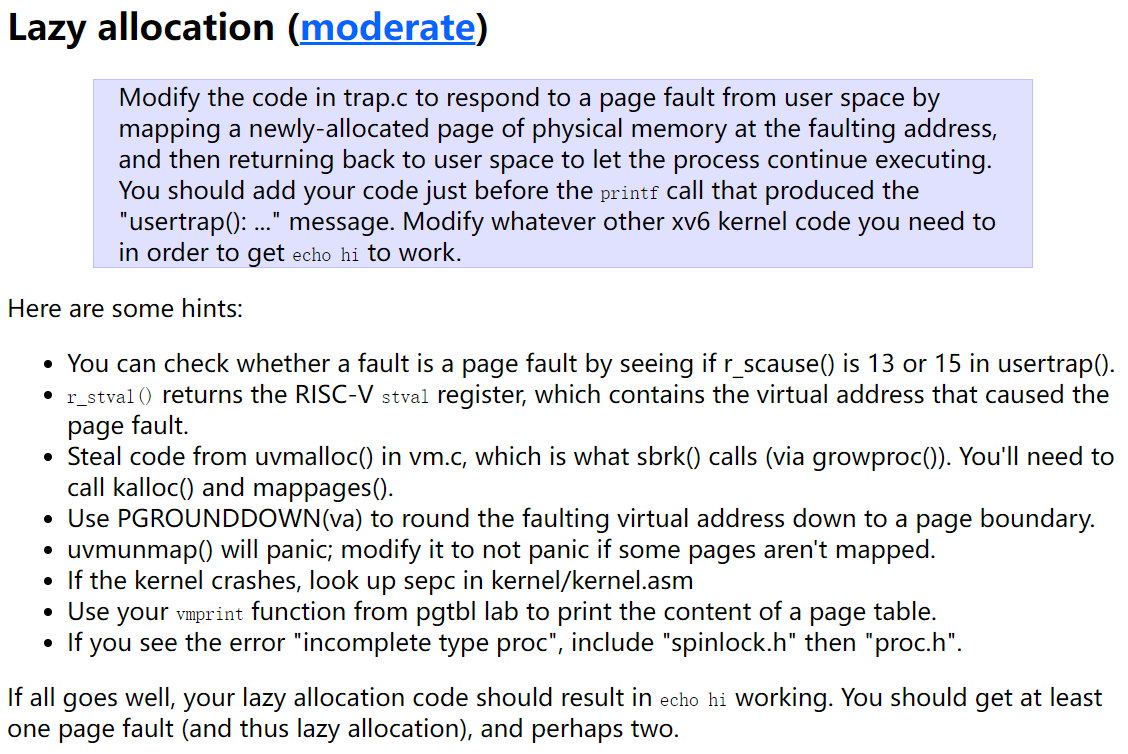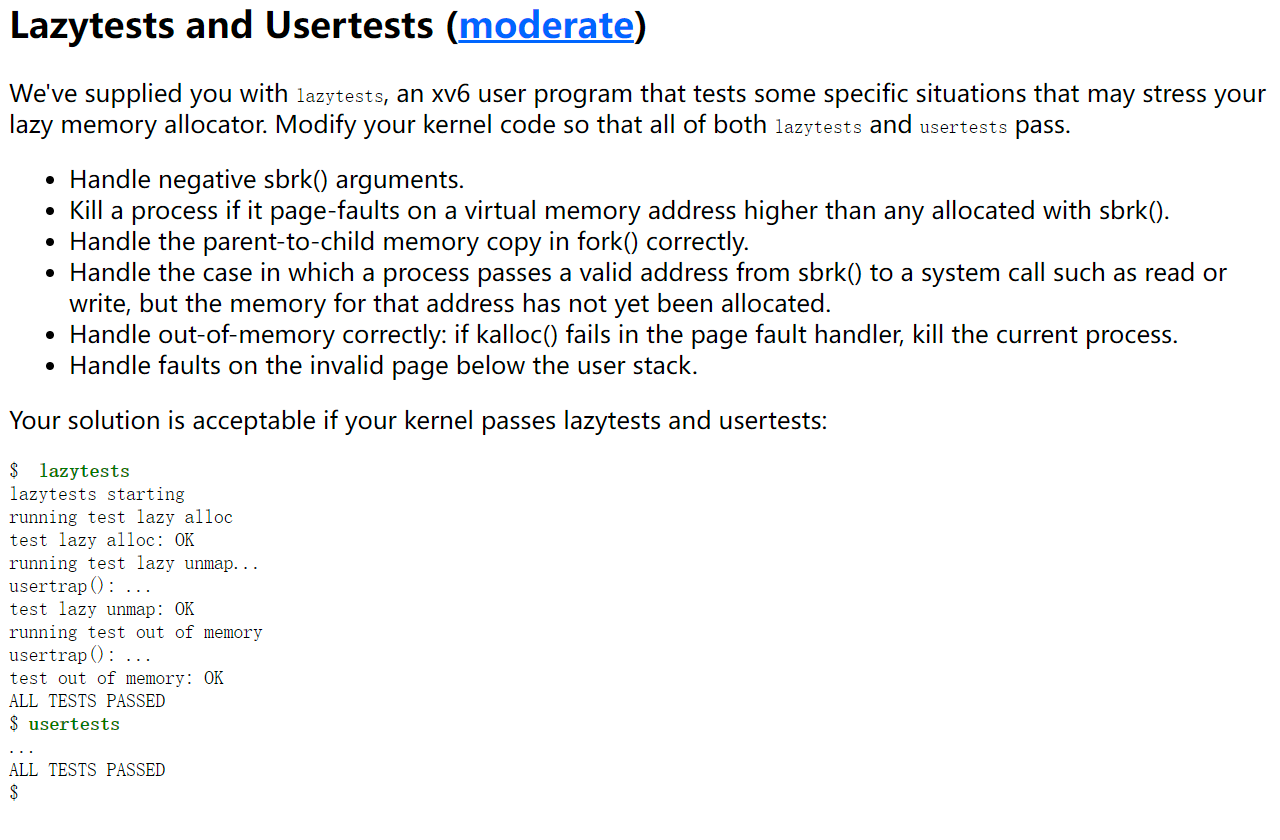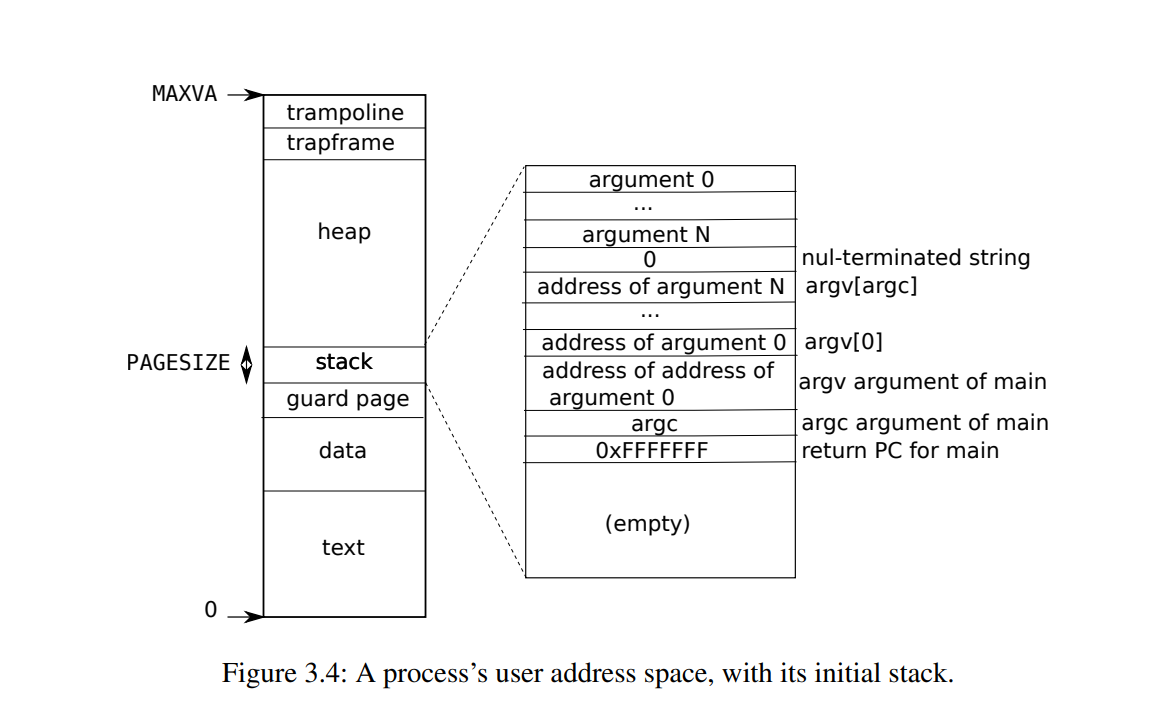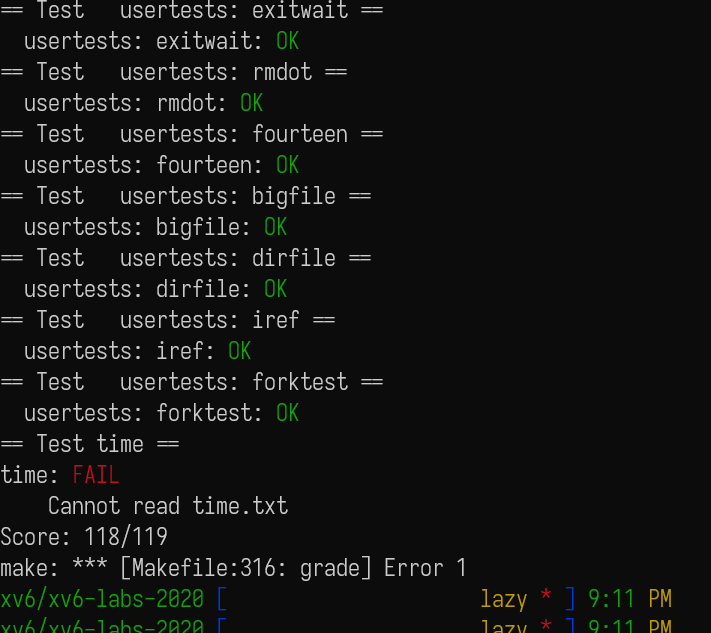upd@2022/9/14：最近把实验的代码放到 github 上了，如果需要参考可以查看这里：

https://github.com/ttzytt/xv6-riscv

# Lab5 (2020): lazy page allocation

## Eliminate allocation from sbrk()uint64
sys_sbrk(void)
{
int n;

if(argint(0, &n) < 0)
return -1;
//   if(growproc(n) < 0) <- 这里删掉实际申请内存的部分
//     return -1;
myproc()->sz += n; // 但是把当前进程占用空间扩大
}

## Lazy allocation……
} else if((which_dev = devintr()) != 0){
// ok
} else if((r_scause() == 13 || r_scause() == 15)){
// do something here
}
else {
printf("usertrap(): unexpected scause %p pid=%d\n", r_scause(), p->pid);
printf("            sepc=%p stval=%p\n", r_sepc(), r_stval());
p->killed = 1;
}
……

int lazy_alloc(uint64 va){
struct proc *p = myproc();
uint64 page_sta = PGROUNDDOWN(va);
uint64* newmem = kalloc();
if(newmem == 0){
return -1;
}
memset(newmem, 0, PGSIZE);
if(mappages(p->pagetable, page_sta, PGSIZE, (uint64)newmem, PTE_W|PTE_R|PTE_X|PTE_U) != 0){
kfree(newmem);
return -1;
}

return 0;
}

void
uvmunmap(pagetable_t pagetable, uint64 va, uint64 npages, int do_free)
{
uint64 a;
pte_t *pte;

if((va % PGSIZE) != 0)
panic("uvmunmap: not aligned");

for(a = va; a < va + npages*PGSIZE; a += PGSIZE){
if((pte = walk(pagetable, a, 0)) == 0)
continue; // 从 panic 改成 continue
// panic("uvmunmap: walk");
// 释放进程的时候会用到 uvmunmap，但是有可能释放的时候这个页根本就没实际被分配
if((*pte & PTE_V) == 0)
continue; // 从 panic 改成 continue
//   panic("uvmunmap: not mapped");
if(PTE_FLAGS(*pte) == PTE_V)
panic("uvmunmap: not a leaf");
if(do_free){
uint64 pa = PTE2PA(*pte);
kfree((void*)pa);
}
*pte = 0;
}
}

## Lazytests and Usertests (moderate)uint64
sys_sbrk(void)
{
int n;
struct proc *p = myproc();
if(argint(0, &n) < 0)
return -1;
if(n < 0){
if(p->sz + n < 0){ // 一个进程不能释放比自己大的空间
return -1;
}
if(growproc(n) < 0){
// 注意这里是实际调用 growproc 去释放空间的。
printf("growproc err\n");
return -1;
}
}else{
myproc()->sz += n;
}
// if(growproc(n) < 0)
//   return -1;
}

Kill a process if it page-faults on a virtual memory address higher than any allocated with sbrk().

int is_lazy_addr(uint64 va){
struct proc *p = myproc();
if(va < PGROUNDDOWN(p->trapframe->sp)
&& va >= PGROUNDDOWN(p->trapframe->sp) - PGSIZE
){
// 防止 guard page，这个之后会提到
return 0;
}
if(va > MAXVA){
return 0;
}
pte_t* pte = walk(p->pagetable, va, 0);

if(pte && (*pte & PTE_V)){
return 0;
}

if(va >= p->sz){
return 0;
}

return 1;
}

……
} else if((which_dev = devintr()) != 0){
// ok
} else if((r_scause() == 13 || r_scause() == 15) && is_lazy_addr(r_stval())){ // 这里加了一个 is_lazy_addr
// 如果是 page fault，那就直接分配内存
p->killed = 1;
}
}
else {
printf("usertrap(): unexpected scause %p pid=%d\n", r_scause(), p->pid);
printf("            sepc=%p stval=%p\n", r_sepc(), r_stval());
p->killed = 1;
}
……

Handle the parent-to-child memory copy in fork() correctly.

int
uvmcopy(pagetable_t old, pagetable_t new, uint64 sz)
{
pte_t *pte;
uint64 pa, i;
uint flags;
char *mem;

for(i = 0; i < sz; i += PGSIZE){
if((pte = walk(old, i, 0)) == 0)
continue;   // 注意这里，panic 改成了 continue。
// panic("uvmcopy: pte should exist");
if((*pte & PTE_V) == 0)
continue;
// panic("uvmcopy: page not present");
pa = PTE2PA(*pte);
flags = PTE_FLAGS(*pte);
if((mem = kalloc()) == 0)
goto err;
memmove(mem, (char*)pa, PGSIZE);
if(mappages(new, i, PGSIZE, (uint64)mem, flags) != 0){
kfree(mem);
goto err;
}
}
return 0;

err:
uvmunmap(new, 0, i / PGSIZE, 1);
return -1;
}

Handle the case in which a process passes a valid address from sbrk() to a system call such as read or write, but the memory for that address has not yet been allocated.

// Copy from user to kernel.
// Copy len bytes to dst from virtual address srcva in a given page table.
// Return 0 on success, -1 on error.
int
copyin(pagetable_t pagetable, char *dst, uint64 srcva, uint64 len)
{
uint64 n, va0, pa0;

while(len > 0){
va0 = PGROUNDDOWN(srcva);
pa0 = walkaddr(pagetable, va0); // 注意这里
if(pa0 == 0)
return -1;
n = PGSIZE - (srcva - va0);
if(n > len)
n = len;
memmove(dst, (void *)(pa0 + (srcva - va0)), n);

len -= n;
dst += n;
srcva = va0 + PGSIZE;
}
return 0;
}

// Look up a virtual address, return the physical address,
// or 0 if not mapped.
// Can only be used to look up user pages.
uint64
{
pte_t *pte;
uint64 pa;

if(va >= MAXVA)
return 0;

pte = walk(pagetable, va, 0);

if(pte == 0)
return 0;
if((*pte & PTE_V) == 0)
return 0;
if((*pte & PTE_U) == 0)
return 0;
pa = PTE2PA(*pte);
return pa;
}

pte_t *
walk(pagetable_t pagetable, uint64 va, int alloc)
{
if(va >= MAXVA)
panic("walk");

for(int level = 2; level > 0; level--) {
pte_t *pte = &pagetable[PX(level, va)];
if(*pte & PTE_V) { // 这里会判断是否为分配过的地址，
// 如果没分配过并且 alloc 参数还为 0，就会返回 0
pagetable = (pagetable_t)PTE2PA(*pte);
} else {
if(!alloc || (pagetable = (pde_t*)kalloc()) == 0)
return 0;
memset(pagetable, 0, PGSIZE);
*pte = PA2PTE(pagetable) | PTE_V;
}
}
return &pagetable[PX(0, va)];
}

// Look up a virtual address, return the physical address,
// or 0 if not mapped.
// Can only be used to look up user pages.
uint64
{
pte_t *pte;
uint64 pa;

if(va >= MAXVA)
return 0;

lazy_alloc(va);
}
pte = walk(pagetable, va, 0);

if(pte == 0)
return 0;
if((*pte & PTE_V) == 0)
return 0;
if((*pte & PTE_U) == 0)
return 0;
pa = PTE2PA(*pte);
return pa;
}

Handle out-of-memory correctly: if kalloc() fails in the page fault handler, kill the current process.

uint64 fault_addr = r_stval();
p->killed = 1;
}

Handle faults on the invalid page below the user stack.if(va < PGROUNDDOWN(p->trapframe->sp)            // 这里使用了用户栈的栈指针 sp 来判断用户栈的虚拟地址
// 因为用户栈的下面就是保护页，所以把
// PGROUNDDOWN(p->trapframe->sp) 当作保护页的上界
&& va >= PGROUNDDOWN(p->trapframe->sp) - PGSIZE
){
return 0;
}